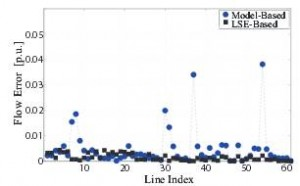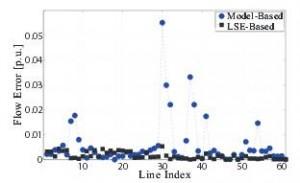Figure 20 (a): Transmission line flow deviation predicted via model- and measurement-based approaches

Christine Chen with adviser A. Domínguez-García

Power system operators perform static security analyses in order to monitor and maintain operational reliability. These studies may include repeated computations, for each credible contingency, of power flow solutions using the full nonlinear power flow model or a linearized model. To reduce the computational burden of evaluating repeated power flow solutions, linear sensitivity distribution factors (DFs), such as injection shift factors (ISFs), are used to predict the effect of an operating point change on the system. In general, these online studies require the maintenance of up-to-date and accurate internal system model and external balancing area equivalents.
Conventional model-based studies are not ideal since the results depend on an accurate model with up-to-date network topology. Therefore, it is important to develop power system monitoring tools that adapt to changes in operating point and topology. Phasor measurement units (PMUs) are an enabling technology for developing measurement-based monitoring tools. We propose a measurement-based method to estimate DFs by finding the solution of a system of linear equations formulated using real power-bus injection and line-flow data obtained from PMU measurements. Specifically, we considered an overdetermined system, with more equations than unknown ISFs, and obtained the solution via linear least-squares errors (LSE) estimation.Figure 20 (b): Transmission line flow deviation predicted via model- and measurement-based approaches with line outages

We illustrate the method’s effectiveness by comparing the DFs obtained via the proposed measurement-based approach to those obtained from the model-based one for the IEEE 118-bus system. Figure 20(a) shows the deviation of transmission line flows predicted via model- and measurement-based approaches from the actual quantities for lines Basedin the internal system. The measurement-based approach yields, on average, more accurate results than the model-based one. In fact, the mean squared error (MSE) for the model-based solution is 0.0066, whereas the measurement-based approach yields an MSE of 0.0015. Suppose line outages have occurred in the external system, unbeknownst to the internal system operators. Again, we compare the errors resulting from the model- and measurement-based approaches against the benchmark nonlinear power flow solution in Fig. 20(b). By visual inspection, we note that the errors in some line flows increase for the model-based approach, while they remain similar to the base case for the measurement-based approach. In fact, the MSE for the post-contingency flows obtained via the model-based approach increases to 0.0086, while those obtained via the measurement-based approach still yield an MSE of 0.0015.

The research was made possible by funding provided by the U.S. Department of Energy for The Future Grid to Enable Sustainable Energy Systems, an initiative of the Power Systems Engineering Research Center